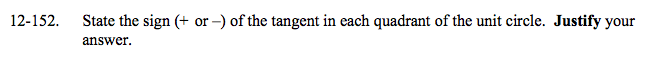### Home > A2C > Chapter 12 > Lesson 12.3.2 > Problem12-152

12-152.

State the sign (+ or −) of the tangent in each quadrant of the unit circle. Justify your answer. Homework Help ✎$\text{Remember tan\theta=\frac{sin\theta}{cos\theta}.}$

Where are the signs of sinθ and cosθ in each quadrant?